UP TO 15 % DISCOUNT

Get Your Assignment Completed At Lower Prices

Plagiarism Free Solutions
100% Original Work
24*7 Online Assistance
Native PhD Experts
Hire a Writer Now
ENG311: Digital Signal Processing Assignment, SUSS Singapore Appraise the Region of Convergence (ROC) of 𝐺𝐺(𝑧𝑧) + 𝐻𝐻(𝑧𝑧) whose individual z transforms are those of causal signals and their ROCs are given as
 University Singapore University of Social Science (SUSS) Subject ENG311: Digital Signal Processing
Posted on: 13th Jun 2023

# ENG311: Digital Signal Processing Assignment, SUSS Singapore Appraise the Region of Convergence (ROC) of 𝐺𝐺(𝑧𝑧) + 𝐻𝐻(𝑧𝑧) whose individual z transforms are those of causal signals and their ROCs are given as

Q1. Appraise the Region of Convergence (ROC) of 𝐺𝐺(𝑧𝑧) + 𝐻𝐻(𝑧𝑧) whose individual z-transforms are those of causal signals and their ROCs are given as: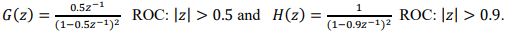(a) |𝑧𝑧| > 0.45
(b) |𝑧𝑧| > 0.5
(c) |𝑧𝑧| > 0.9
(d) 0.5 < |𝑧𝑧| < 0.9
(e) 0.5 < |𝑧𝑧| < 4.5

Q2. Identify the TRUE statement below.
(a) A discrete-time system if evaluated to be dynamic is said to possess memory.
(b) A linear system is one that violates the superposition principle.
(c) A system is time invariant if its input-output characteristics change with
time.
(d) Unstable systems usually exhibit erratic and extreme behaviour but seldom cause overflow
(e) A causal system is said to be one where the output at any time, n, depends on past, present and future inputs.

Q3. The one-sided exponential sequence,  17 𝑛𝑛 , 𝑛𝑛 ≥ 0, will have its sum of components, ∑  1 17 𝑛𝑛 ∞ 𝑛𝑛=0 converging to:Q4. Select the element that describes the picture of Figure Q4 below.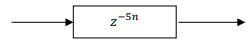(b) Quintuple Unit Delay Element
(d) 5n samples delay element
(e) n-Unit Delay Element
where 𝑛𝑛 is an integer

Q5. A digital video camera captures an image signal onto its CMOS sensor plate with the following characteristics:
(a) One-dimensional, multichannel, discrete-time
(b) Multi-dimensional, single channel, continuous-time
(c) Multi-dimensional, single channel, discrete-time
(d) One dimensional, single channel, discrete-time
(e) One dimensional, multi-channel, continuous-time

Q6. An amplitude modulated (AM) waveform has a spectrum shown in Figure Q6. Debate the optimum sampling frequency that should be employed should the AM signal be first digitized then sent over a digital network?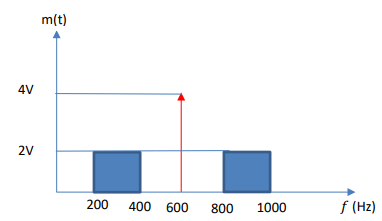(a) The sampling frequency should be at least 2 kHz.
(b) The sampling frequency can be greater than 2 kHz.
(c) The sampling frequency should be at least twice the highest frequency of the upperside band.
(d) The sampling frequency should be greater than highest frequencies of both the lower and upper sidebands by such a margin so as to avoid frequency aliasing.
(e) Any of the above sampling strategies will work

Q7. Construct the assumed form of the particular solution to the following second order difference equation:
𝑦𝑦[𝑛𝑛] − 3𝑦𝑦[𝑛𝑛 − 1] − 10𝑦𝑦[𝑛𝑛 − 2] = 𝑥𝑥[𝑛𝑛] + 6𝑥𝑥[𝑛𝑛 − 1],
when the following input sequence is applied: 𝑥𝑥[𝑛𝑛] = 5𝑛𝑛𝑢𝑢[𝑛𝑛],
(a) 𝑦𝑦𝑝𝑝[𝑛𝑛] = 𝐶𝐶3𝑛𝑛(5)𝑛𝑛𝑢𝑢[𝑛𝑛]
(b) 𝑦𝑦𝑝𝑝[𝑛𝑛] = 𝐶𝐶1(1)𝑛𝑛 + 𝐶𝐶2(2)𝑛𝑛
(c) 𝑦𝑦𝑝𝑝[𝑛𝑛] = 𝐶𝐶1(−2)𝑛𝑛 + 𝐶𝐶2(5)𝑛𝑛 + 𝐶𝐶3𝑛𝑛(5)𝑛𝑛𝑢𝑢[𝑛𝑛]
(d) 𝑦𝑦𝑝𝑝[𝑛𝑛] = 𝐶𝐶3(5)𝑛𝑛𝑢𝑢[𝑛𝑛]
(e) 𝑦𝑦𝑝𝑝[𝑛𝑛] = 𝐶𝐶1(−2)𝑛𝑛 + 𝐶𝐶2(5)𝑛𝑛
Noting that 𝐶𝐶1, 𝐶𝐶2 and 𝐶𝐶3 are arbitrary constants.

Q8. Select from the choice(s) below, the type of time-domain signal whose frequency spectra is given by the following analysis equation: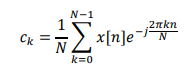(a) Continuous-time and periodic signal
(b) Discrete-time and periodic signal
(c) Discrete-time and periodic spectrum
(d) Continuous-time and aperiodic signal
(e) Discrete frequency and aperiodic spectrum

Q9. Solve for the complex spectrum of the following signal, as a function of frequency, f

Q10. Evaluate the period of the following discrete-time signal:(a) 210 samples
(b) 105 samples
(c) 7 samples
(d) 5 samples
(e) 3 samples

Q11. Appraise the Region of Convergence (ROC) of 𝐺𝐺(𝑧𝑧) + 𝐻𝐻(𝑧𝑧) whose individual z-transforms are those of causal signals and their ROCs are given as: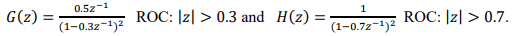(a) |𝑧𝑧| > 0.21
(b) |𝑧𝑧| > 0.3
(c) 0.3 < |𝑧𝑧| < 4.9
(d) 0.3 < |𝑧𝑧| < 0.7
(e) |𝑧𝑧| > 0.7

Q12. Identify the TRUE statement below.
(a) A discrete-time system if evaluated to be dynamic is said to possess no memory.
(b) A linear system is one that obeys the superposition principle.
(c) A system is time-invariant if its input-output characteristics change with time occasionally, but otherwise remain the same.
(d) Unstable systems usually exhibit orderly behavior and seldom cause
overflow
(e) A causal system is said to be one where the output at any time, n, depends on present and future inputs.

Q14. Select the element that describes the picture of Figure Q14 below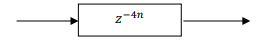(d) 4n samples delay element
(e) n-Unit Delay Element
where 𝑛𝑛 is an integer.

Q15. A Dolby THX encoded system captures a discrete-time signal which is:
(a) Multi-dimensional, multichannel, discrete time
(b) Multi-dimensional, single channel, continuous-time
(c) Multi-dimensional, multi channel, continuous-time
(d) One dimensional, single channel, discrete-time
(e) One dimensional, multi-channel, continuous-time

Q16. An amplitude modulated (AM) waveform has a spectrum shown in Figure Q16. Debate the optimum sampling frequency that should be employed should the AM signal be first digitized then sent over a digital network?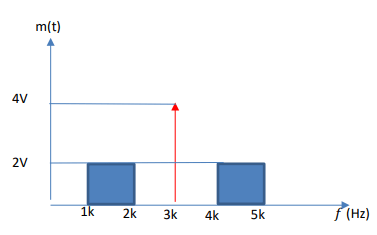(a) The sampling frequency should be at least 10 kHz.
(b) The sampling frequency can be greater than 10 kHz.
(c) The sampling frequency should be at least twice the highest frequency of the upperside band.
(d) The sampling frequency should be greater than highest frequencies of both the lower and upper sidebands by such a margin so as to avoid frequency aliasing.
(e) Any of the above sampling strategies will work.

Q17. Construct the assumed form of the total solution to the following second order difference equation:

Q18. Select from the choice(s) below, the type of time-domain signal whose the following analysis equation gives frequency spectra:(a) Discrete-time and periodic signal
(b) Continuous-time and periodic signal
(c) Discrete-time and periodic spectrum
(d) Continuous-time and aperiodic signal
(e) Discrete frequency and aperiodic spectrum

Q19. Solve for the magnitude spectrum of the following signal, as a function of frequency, f

Q20. Evaluate the period of the following discrete-time signal:

Q21. A stereophonic (2-channel) audio system with a dynamic range of 10 volts recorded an hour long music album using a 24 bit, 44,1 kHz analogue-to-digital converter.

Determine the bit rate and the highest frequency component that can be sampled without aliasing as well as the resolution of the stored signals. What is the total data stored in the disk in bytes?

Q22 (a) Write down an expression in terms of z of a discrete-time transfer function containing 9 zeros located at the origin and 7 poles equally spaced out in angular distance at a radial distance of 0.7 units from the origin.

Q23. A tri-channel seismic sensor with a dynamic range of 10 volts recorded amplitude vibration signals at 40 samples per second using a 12-bit linear quantization analog-to-digital converter. Determine the bit rate and the
highest frequency component that can be sampled without aliasing as well as the resolution of the stored signals. What is the hourly data rate sent?

Q24 (a) Write down an expression in terms of z of a discrete-time transfer a function containing 5 poles located at the origin and 8 zeros equally spaced out in angular distance along the unit circle.

Get Help By Expert

Calling all Singapore students studying at Singapore University of Social Sciences (SUSS) and pursuing ENG311: Digital Signal Processing! Struggling with your final year exam assignments? Don't worry, we've got you covered. Our platform offers the best assignment help in Singapore, providing expert assistance for your coursework. Whether it's analyzing the Region of Convergence (ROC) of complex signals or completing other challenging tasks, our professionals are here to support you. With our reliable Report Writing Service, you can rest assured that your assignments will be delivered with excellence. Take the stress out of your studies and pay our experts for the assistance you need to succeed!

Categories:-##### No Need To Pay Extra
• Turnitin Report

\$10.00

\$9.00
Per Page
• Consultation with Expert

\$35.00
Per Hour
• Live Session 1-on-1

\$40.00
Per 30 min.
• Quality Check

\$25.00
##### Free

New Special Offer

## Get 30% Off

UP TO 15 % DISCOUNT

Get Your Assignment Completed At Lower Prices

Plagiarism Free Solutions
100% Original Work
24*7 Online Assistance
Native PhD Experts
Hire a Writer Now
My Assignment Help SG Services
My Assignment Help SG